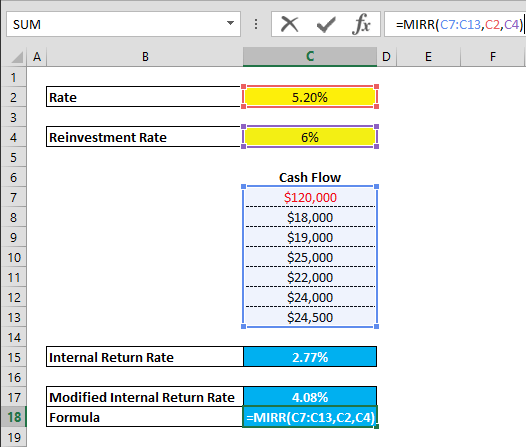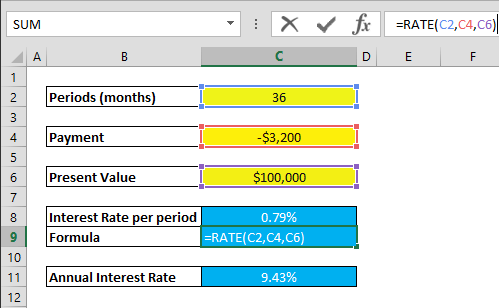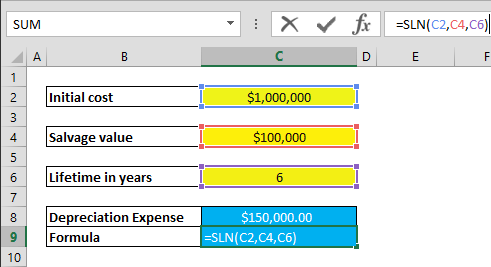Excel is the perfect tool for financial modeling. Many organizations can efficiently create and publish their models using only this tool. It’s no surprise Excel offers a ton of formulas that can handle almost any financial operation.

In this article, we’re going to walk you through the 10 most useful formulas that are vital to the world of financial modeling. This should be a great start, or refresher! Please feel free to download our sample workbook below.

## XNPV

In finance, the Net Present Value (NPV) is a measurement of profit. It is defined as the difference between the present value of cash inflows and the present value of cash outflows over a period of time.

Although Excel has a function named NPV for calculating the net present value for a series of periodic cash flows, this is typically not the case in real world applications. XNPV can work with specific dates of cash flows and therefore is more comprehensive.

### Syntax

=XNPV(rate, values, dates)

### Example## FV

This function answers a simple question, how much money will you have in the future? The FV (Future Value) function calculates the value of a current asset after a specified period of time, based on regular payments and an assumed rate of growth over time.

### Syntax

= FV(rate, nper, pmt, [pv], [type])

### ExampleNote: Optional type argument is omitted in the example above. This variable defines whether payments are due at the beginning of the period or at the end. If type is omitted, it is assumed that payments are due at the end of each period.

## XIRR

Internal rate of return (IRR) is a metric to estimate the profitability of potential investments. The term ‘internal’ refers to the fact that it does not involve external factors, such as inflation or the cost of capital.

Similar to the NPV case, Excel has a function named IRR for calculating the internal return rate for periodic series of cash flows. To calculate the internal rate of return for varying dates of cash flows, use the XIRR function.

### Syntax

= XIRR(values, dates, [guess])

### ExampleNote: Optional type argument is omitted in the example above. This parameter defines a number you think is close to the result. If omitted, it is assumed to be 10%.

## MIRR

The MIRR function represents Modified Internal Rate of Return which is another variation of Internal Rate of Return as the name suggests. The Modified Internal Rate of Return is used in capital budgeting to rank alternative investments of equal size. This method assumes that the investment is profitable and is used in business by using both the cost of the investment and the interest received by reinvesting the cash. Essentially the MIRR function returns the modified internal rate of return for a series of periodic cash flows.

### Syntax

= MIRR(values, finance_rate, reinvest_rate)

### Example## PMT

The PMT (payment) is one of the most well-know function in financial modeling. It returns payment values for a loan with constant payments, and a constant interest rate. Note that the payment returned by PMT includes principal and interest.

### Syntax

= PMT(rate, nper, pv, [fv], [type])

### ExampleNote: You may have noticed that calculated payment amount is negative. This is because of the direction the money is going. Removing the dash (-) or multiplying the result with ‘-1’ returns value into positive.

## PPMT

PPMT is a variation of the PMT function. This function calculates the payment on the principal for a given period for an investment with periodic constant payments and a constant interest rate. It has an additional per period argument (per) which is the period for which the principal is to be calculated. The per argument must be between 1 and nper.

### Syntax

=PPMT(rate, per, nper, pv, [fv], [type])

### Example

The example below shows the PPMT for the 10th month of total 36 months payment period.## EFFECT

The EFFECT is another function in financial modeling and returns the effective annual interest rate, which is the calculating the annual interest rate by adding the effects of compounding in a given period. In finance, interest rates are almost always annual. However, if interest rate compounds in smaller periods, the final interest rate will be higher than the stated annual interest rate.

For example, if a 12.0% annual interest rate compounds monthly, at the end of the year, effective interest rate will become 12.7% because of 1.0% compounding each month.

The NOMINAL function can be used to calculate annual interest rate by given effective annual interest rate and period. Effectively, going the other way around.

### Syntax

=EFFECT(nominal_rate, npery)

### Example## RATE

The RATE function returns the interest rate per period of an annuity. It can calculate the interest rate required to pay off the loan in full, for a given period of time.

### Syntax

=RATE(nper, pmt, pv, [fv], [type], [guess])

### ExampleNote 1: The payment value is entered as a negative value, because of the direction the money is going (i.e. out of pocket)

Note 2: The result represents the interest rate per period (i.e. month). Multiply the result with 12 to find the annual interest rate.

## DB

DB stands for Declining Balance. This function calculates the depreciation expense of an asset for a specified period, using the ‘fixed-declining balance’ method.

Excel uses different calculations while calculating depreciation values at different periods. The rate is assumed to be 1 - ((salvage / cost) ^ (1 / life)) and is rounded to three decimal places.

• first period,

cost * rate * month / 12

• following periods,

(cost - total depreciation from prior periods) * rate

• last period,

((cost - total depreciation from prior periods) * rate * (12 - month)) / 12

### Syntax

=DB(cost, salvage, life, period, [month])

### ExampleNote: The omitted Month argument is assumed to be 12.

## SLN

The SLN (straight-line) function calculates the ‘straight-line’ depreciation of an asset for one period. This method assumes that the cost of a fixed asset is reduced uniformly over the useful lifespan of the asset.

The straight-line method is calculated like this:

(initial cost – salvage value) / lifetime

### Syntax

=SLN(cost, salvage, life)

### ExampleWe covered 10 most common and useful formula in financial modeling, but go ahead and experiment yourself! We hope this was a good place to start, or a refresher, to dive into doing finance with Excel!